# RS Aggarwal Solutions for Class 10 Maths Chapter 3 Linear Equations in Two Variables Exercise 3E Page number 145

R S Aggarwal Solutions for Class 10 Maths Chapter 3 Linear Equations in Two Variables, contains solutions for exercise 3E questions. This set contains answers to all the questions present in this exercise. R S Aggarwal solutions on exercise 3e is all about word problems on linear equations and each answer has been prepared by the subject experts at BYJU’S. Download  RS Aggarwal Solutions of Class 10  for free to practice them offline.

## Download PDF of RS Aggarwal Solutions for Class 10 Maths Chapter 3 Linear Equations in Two Variables Exercise 3E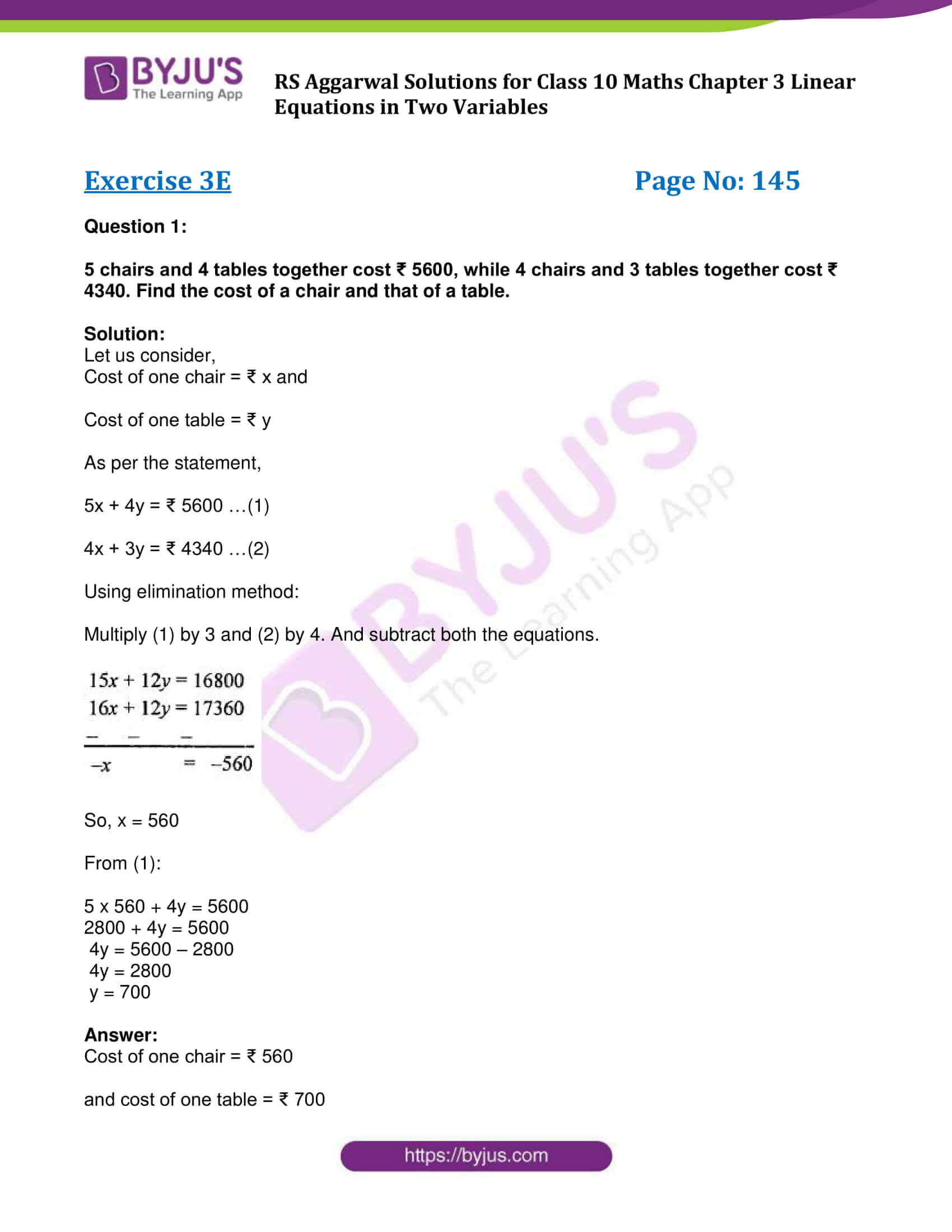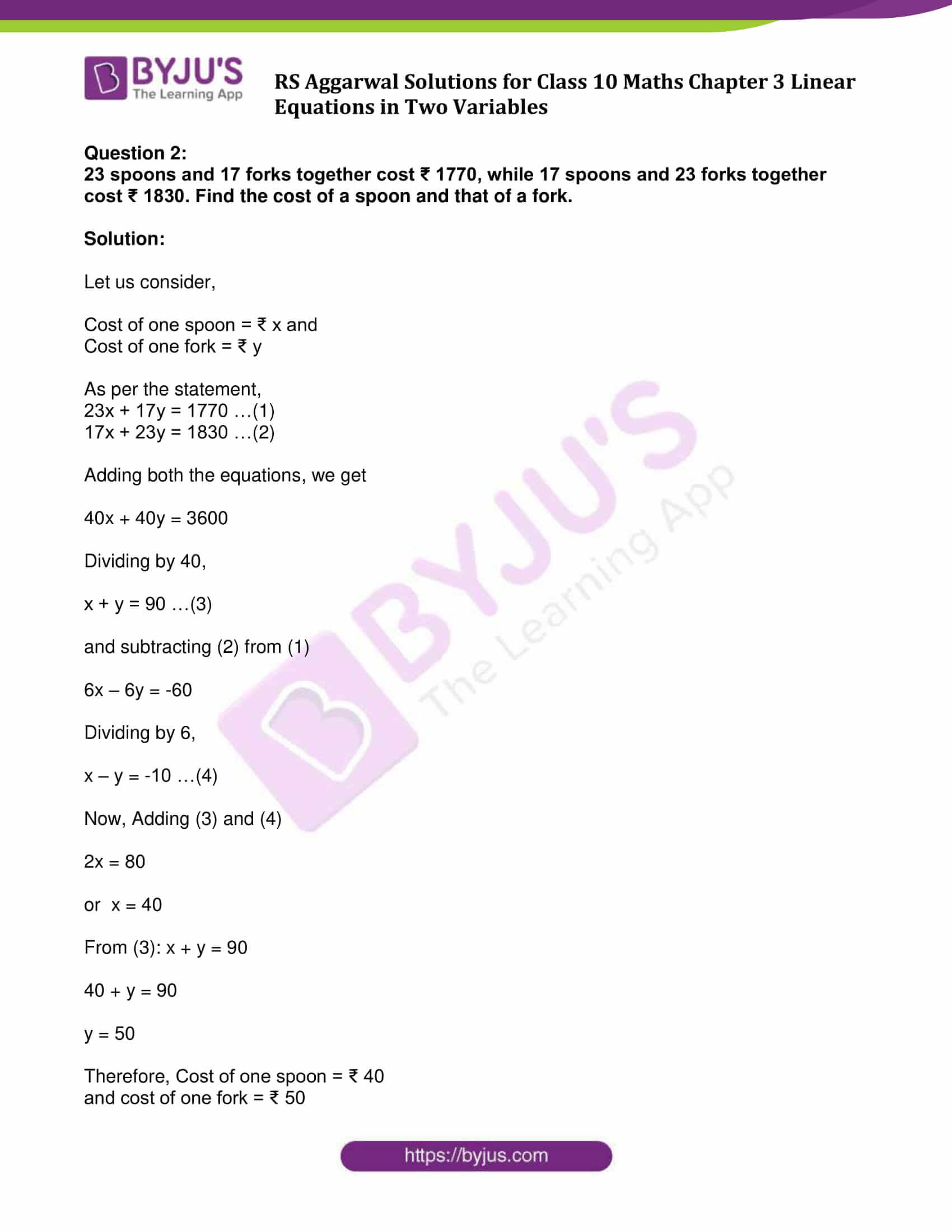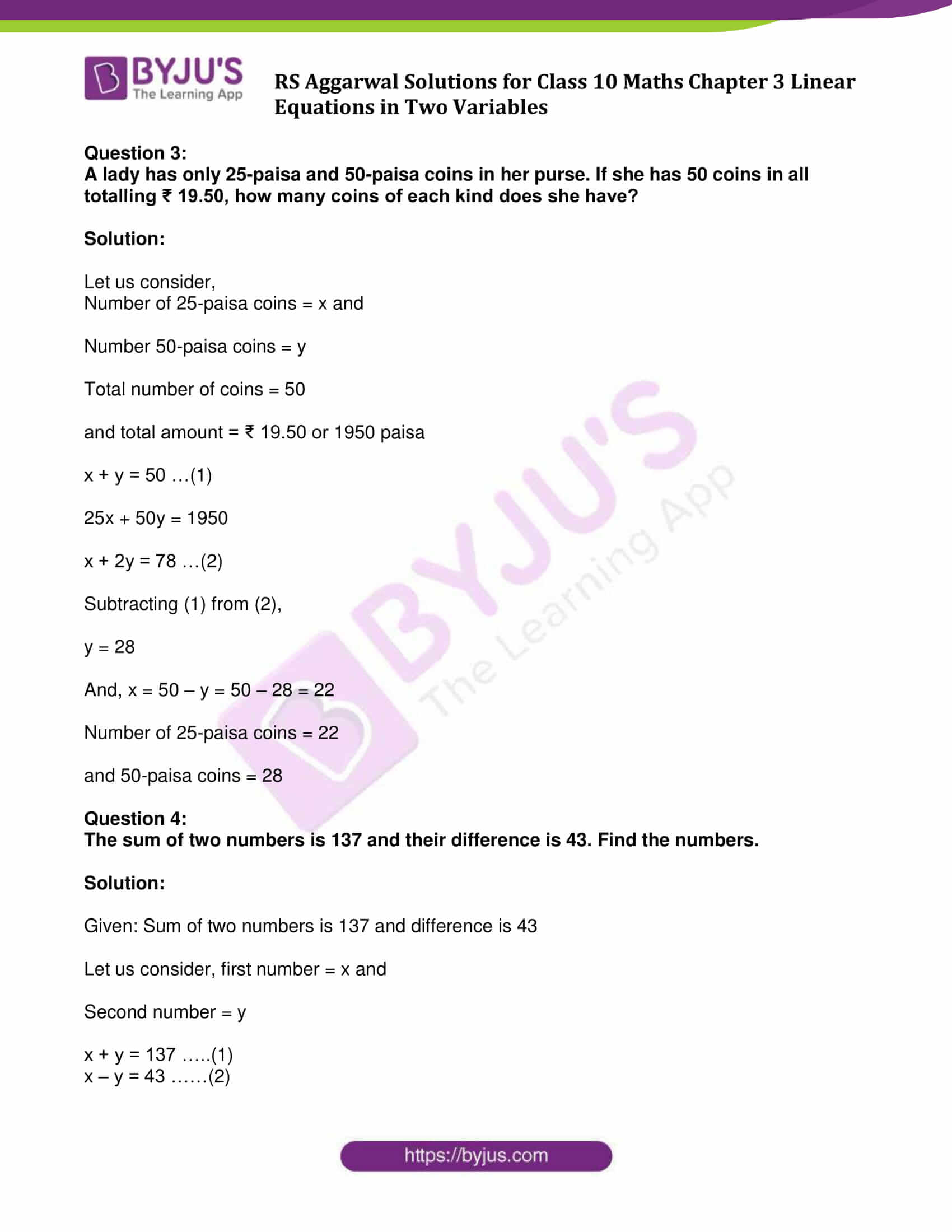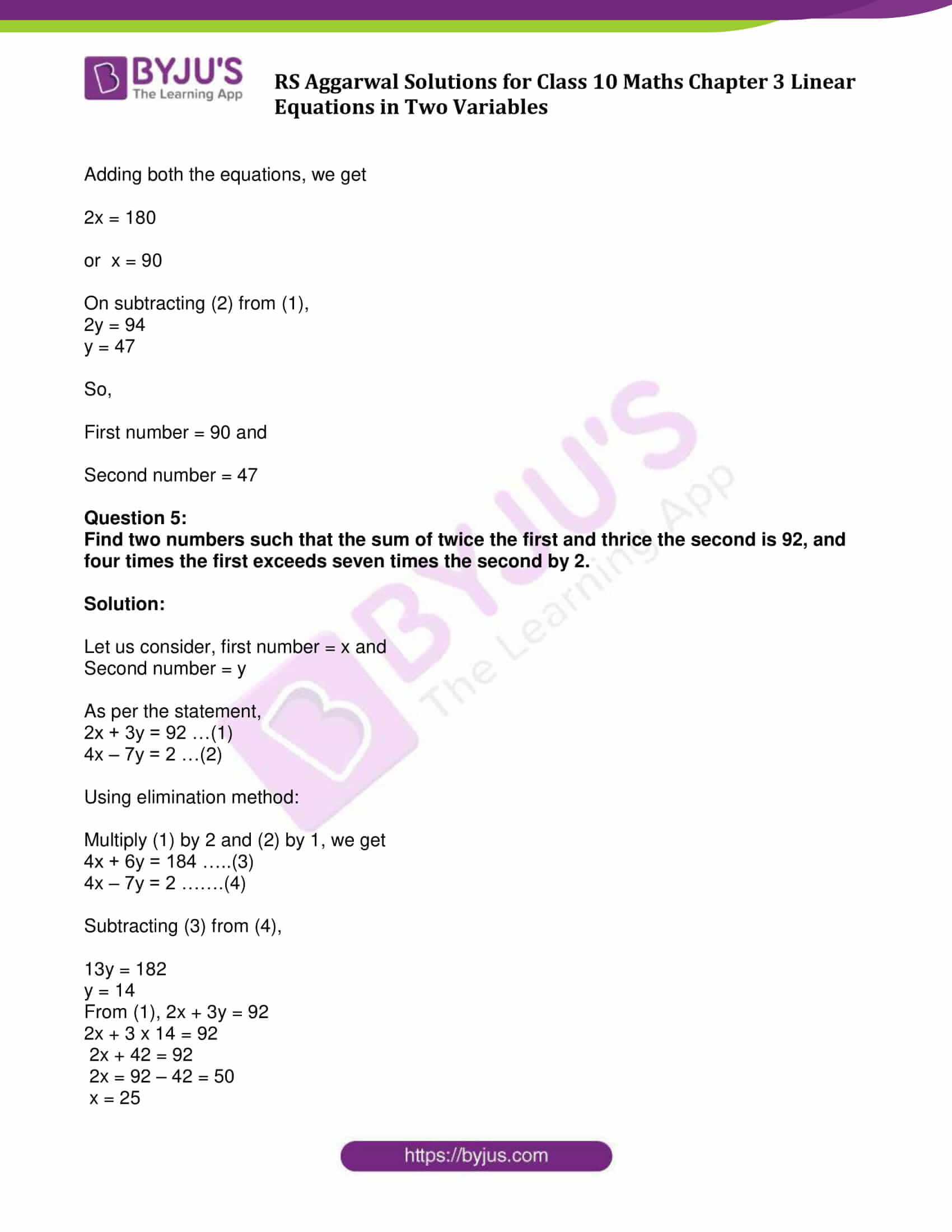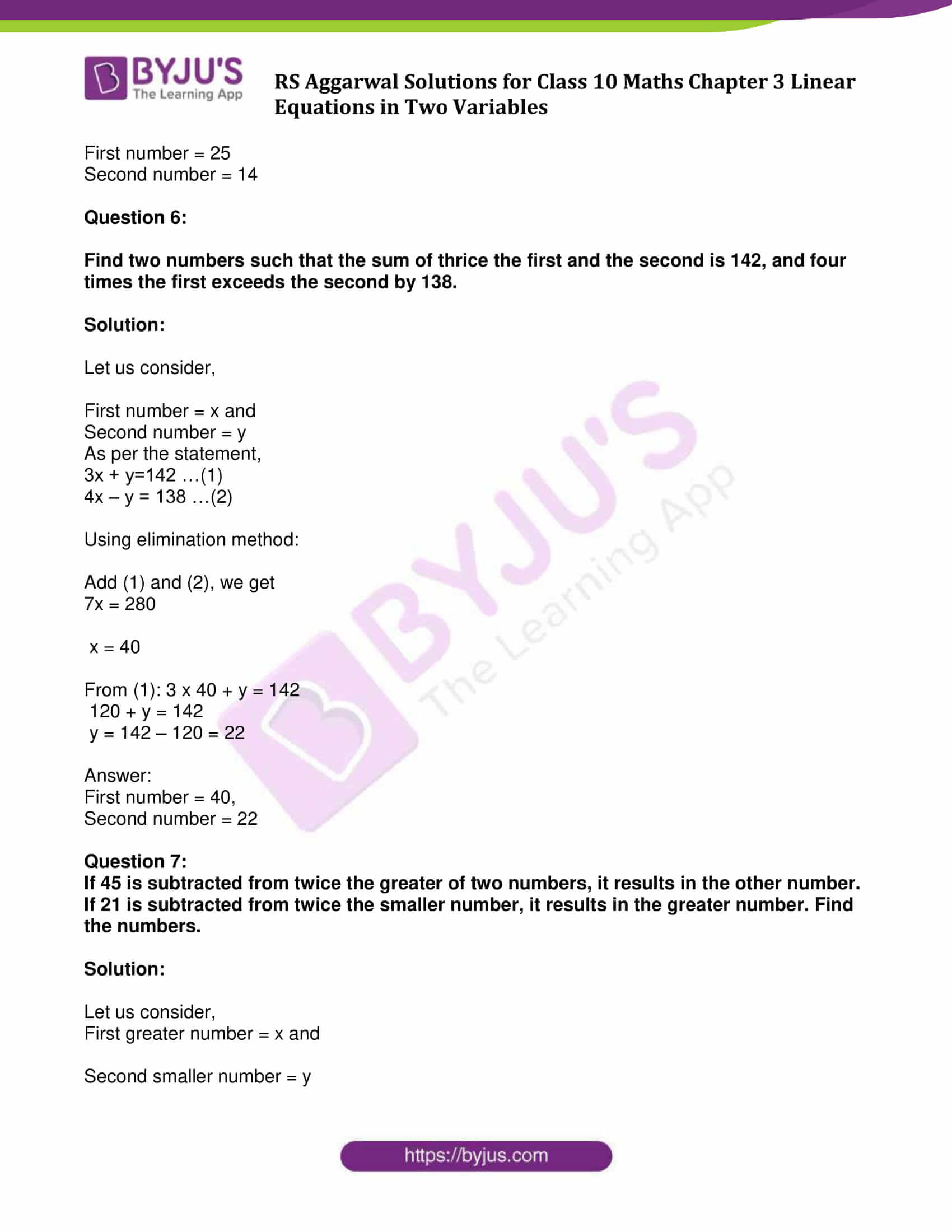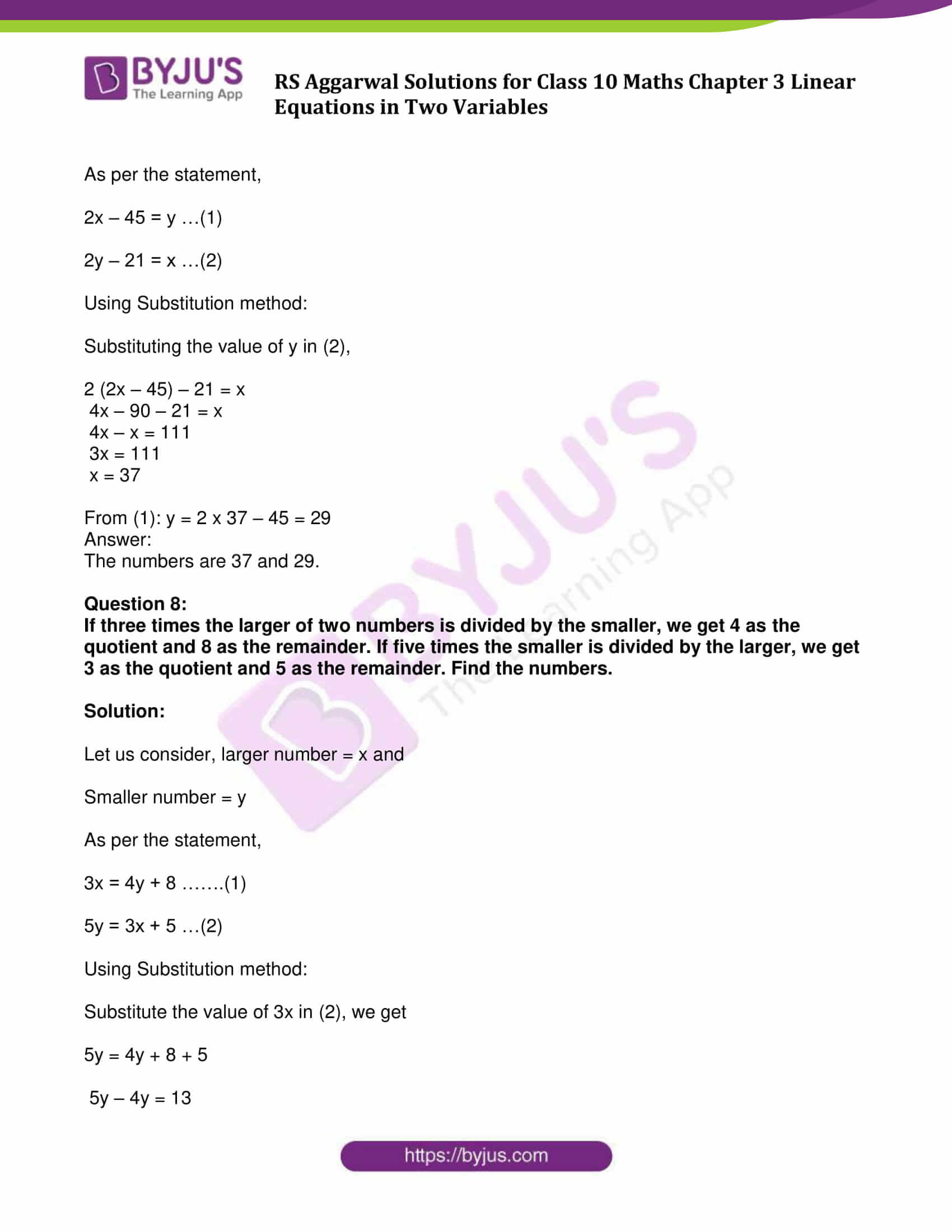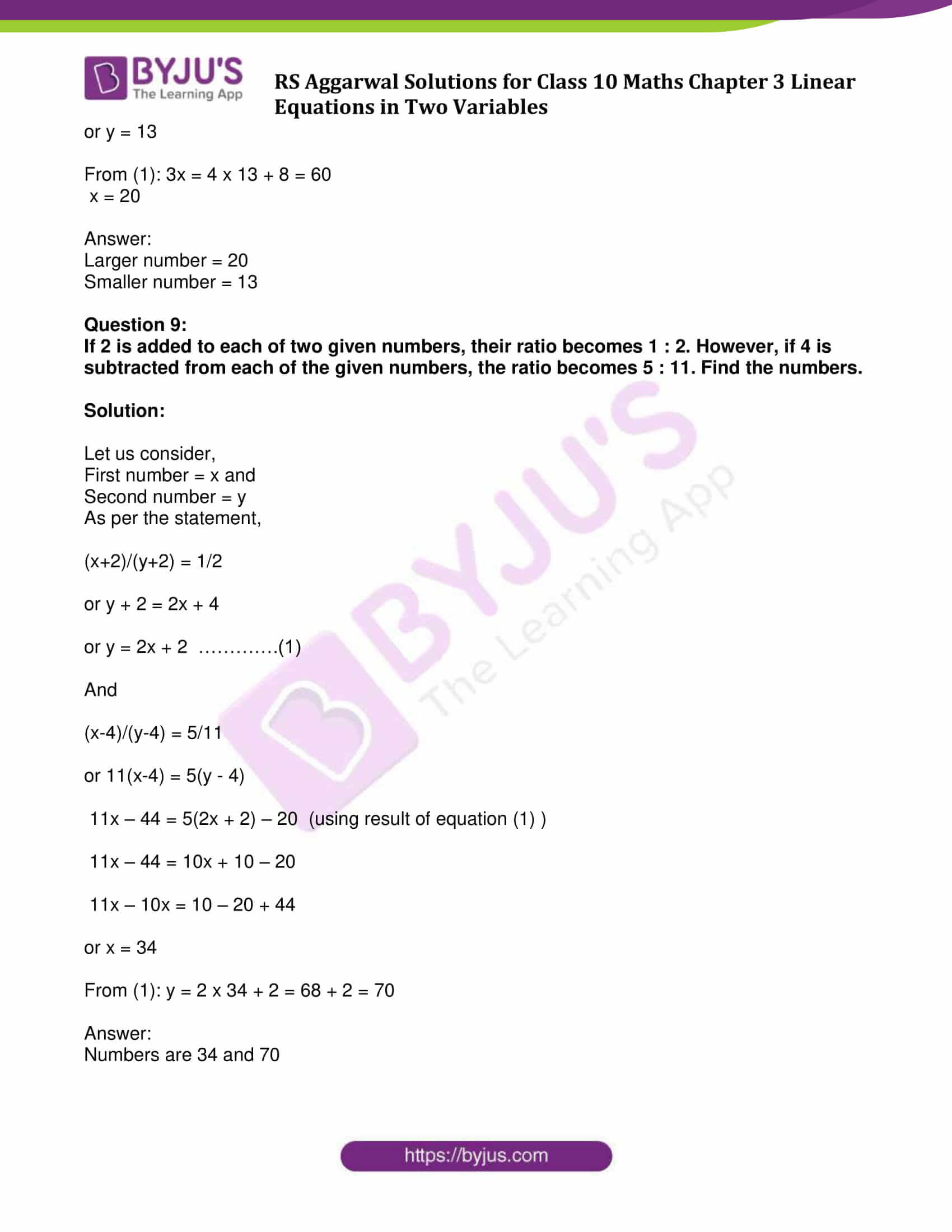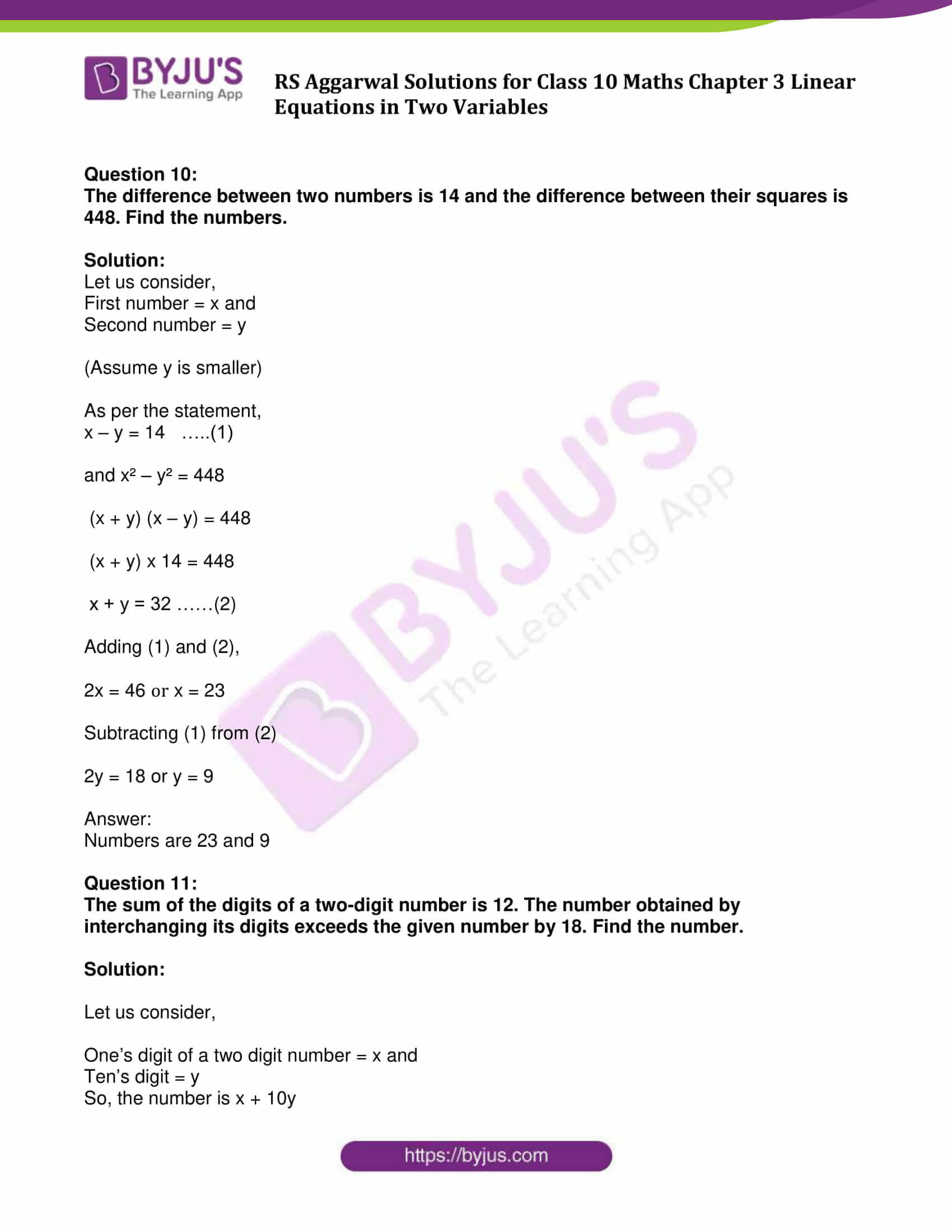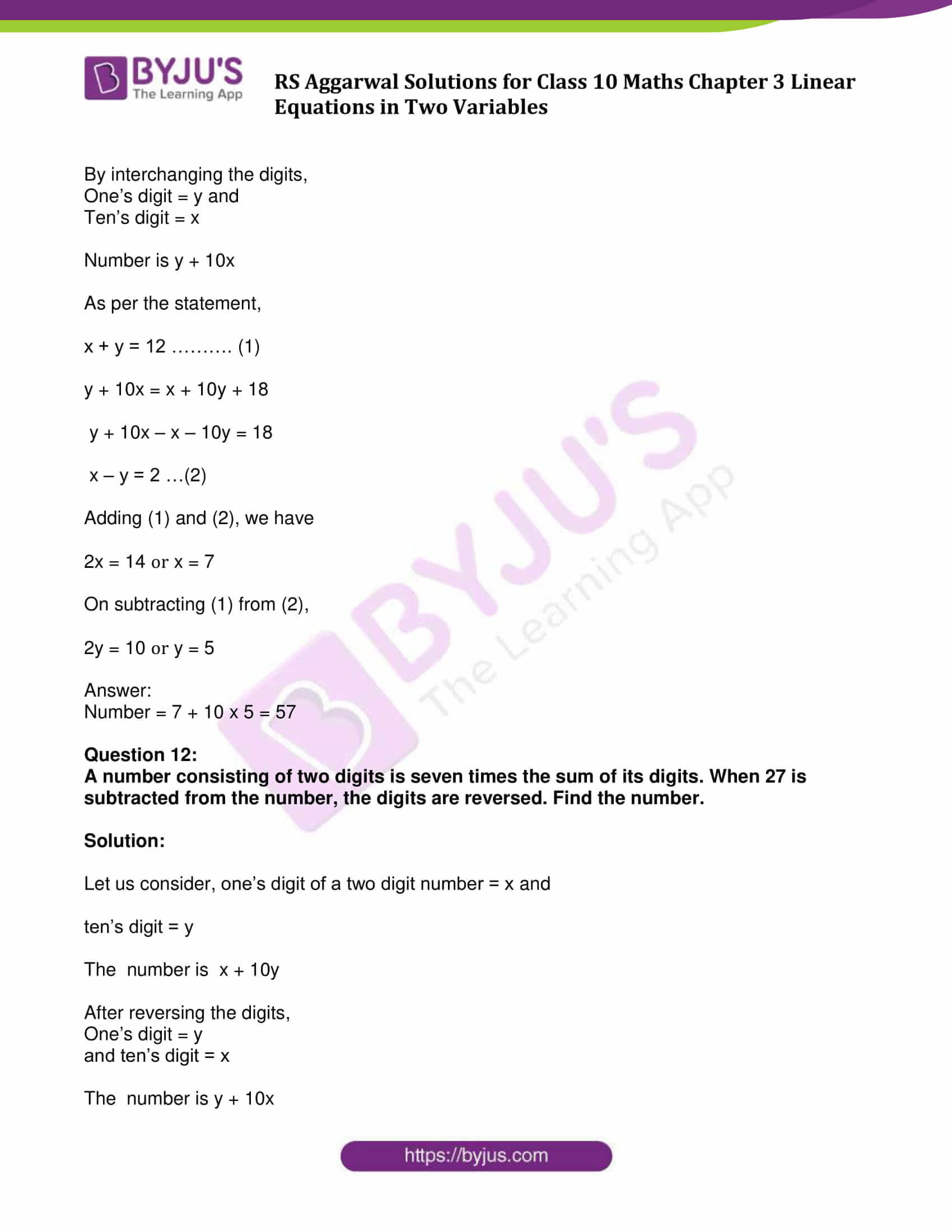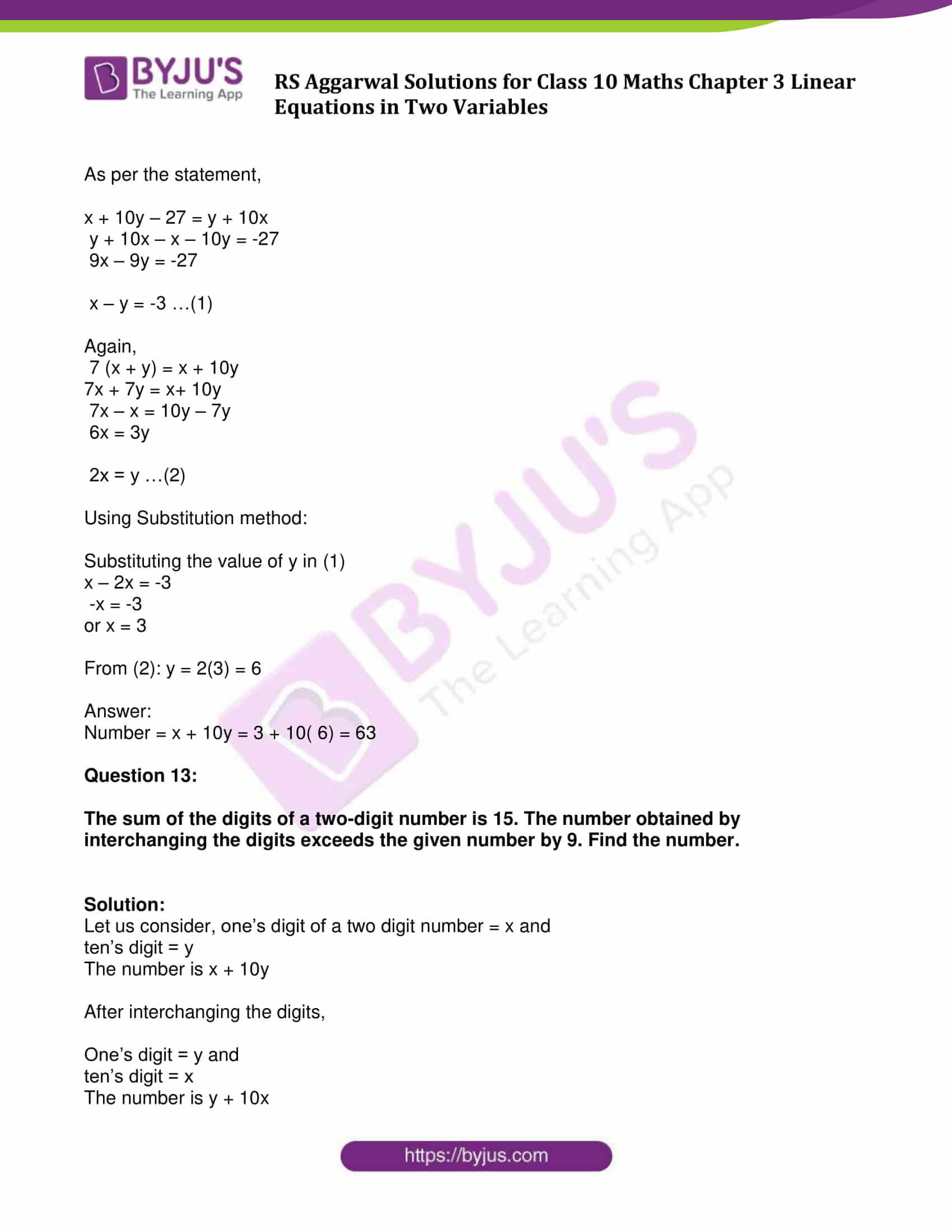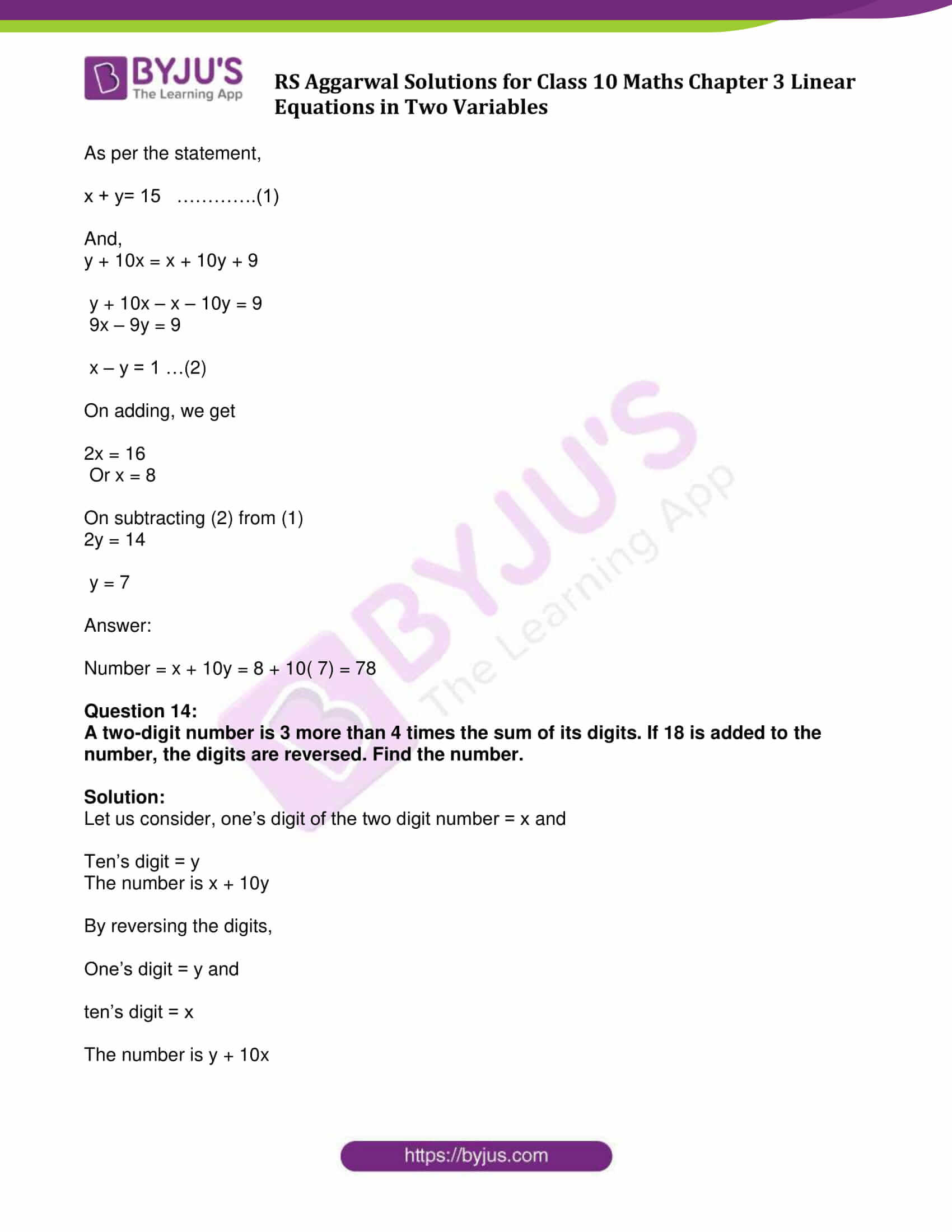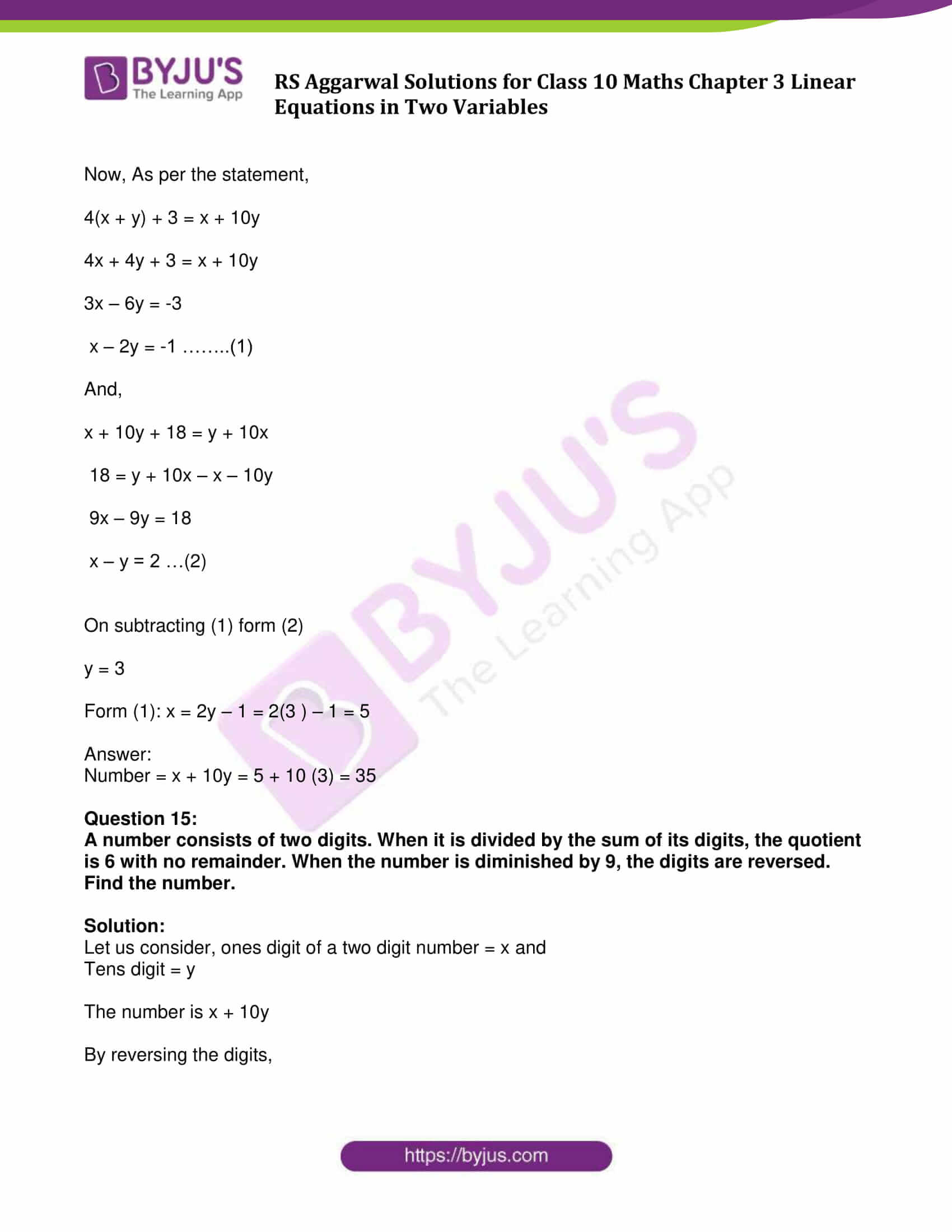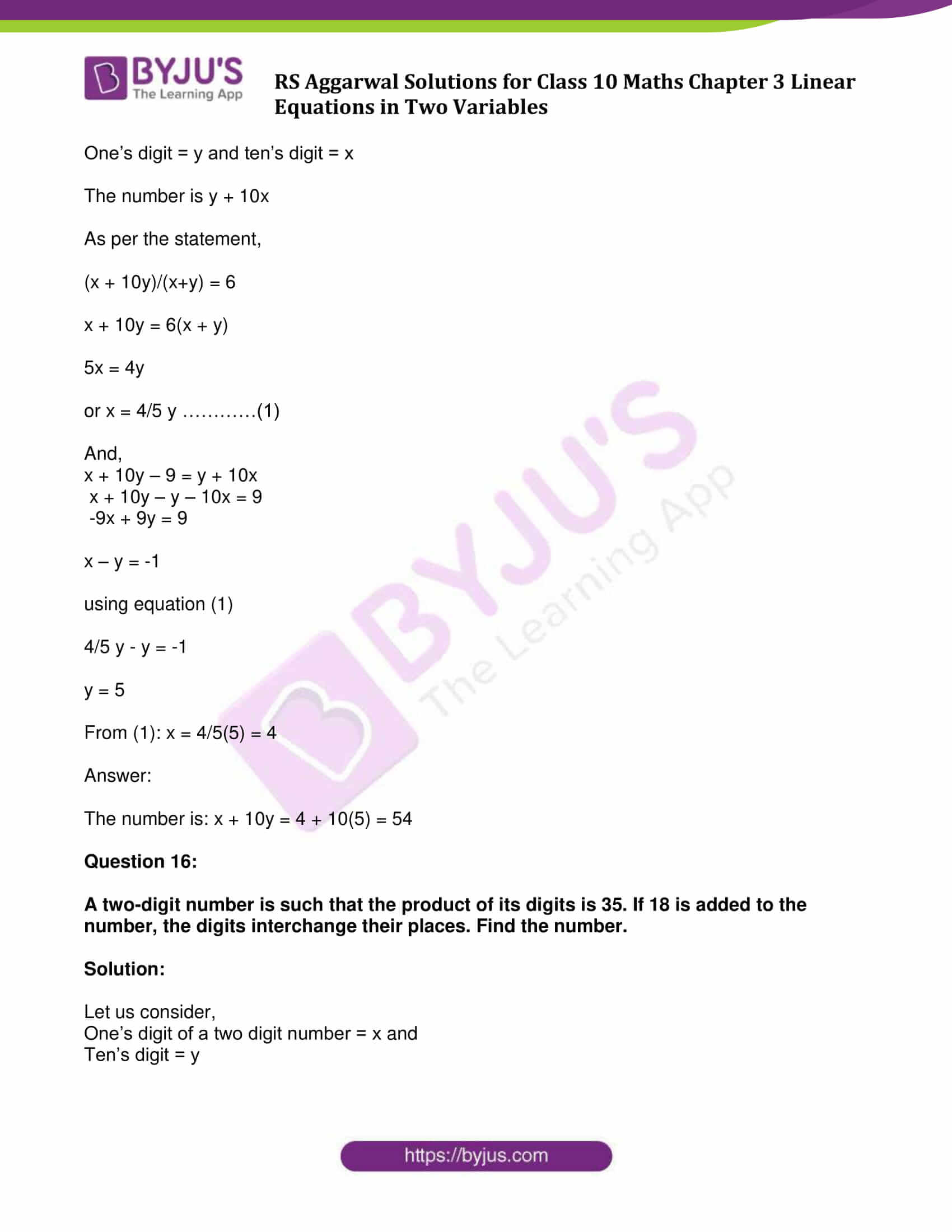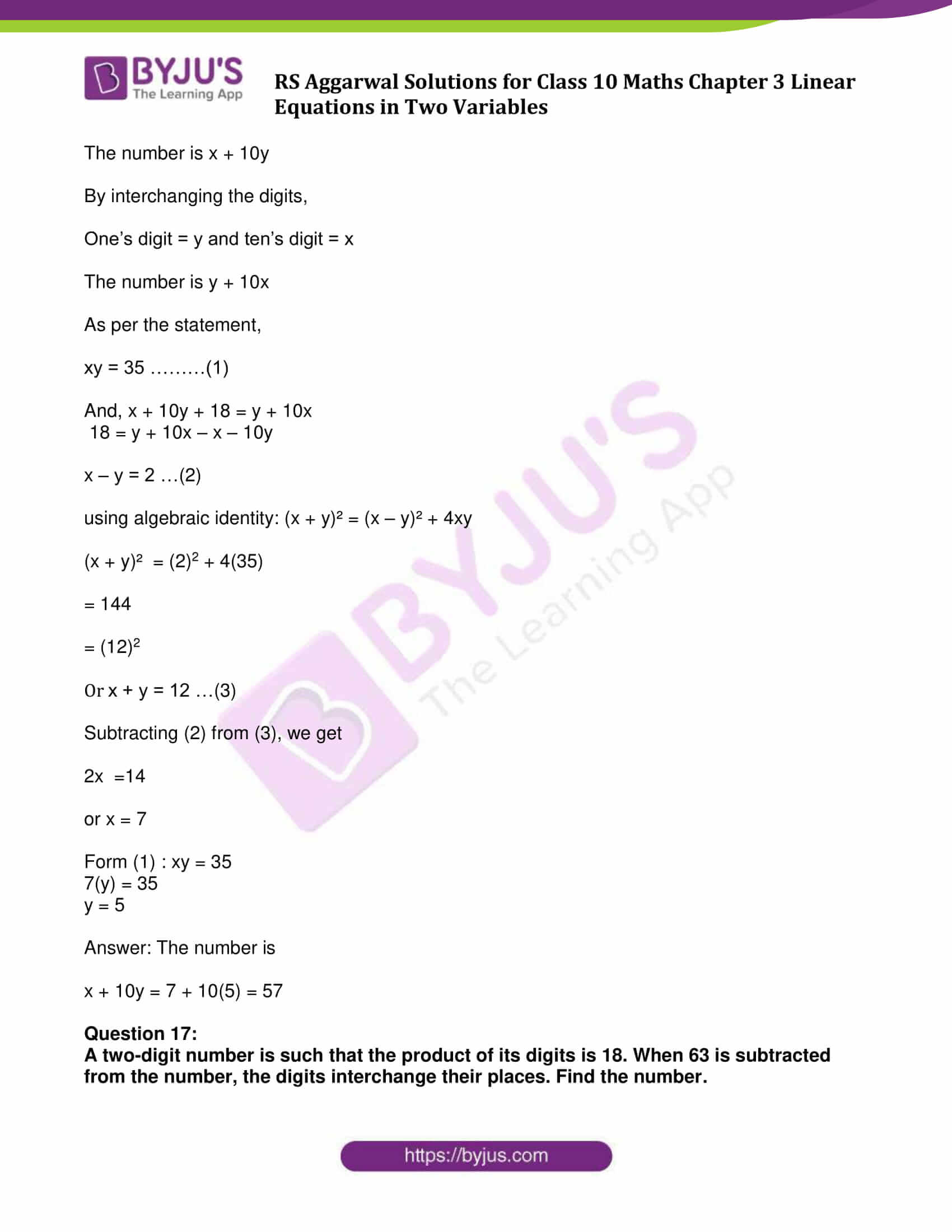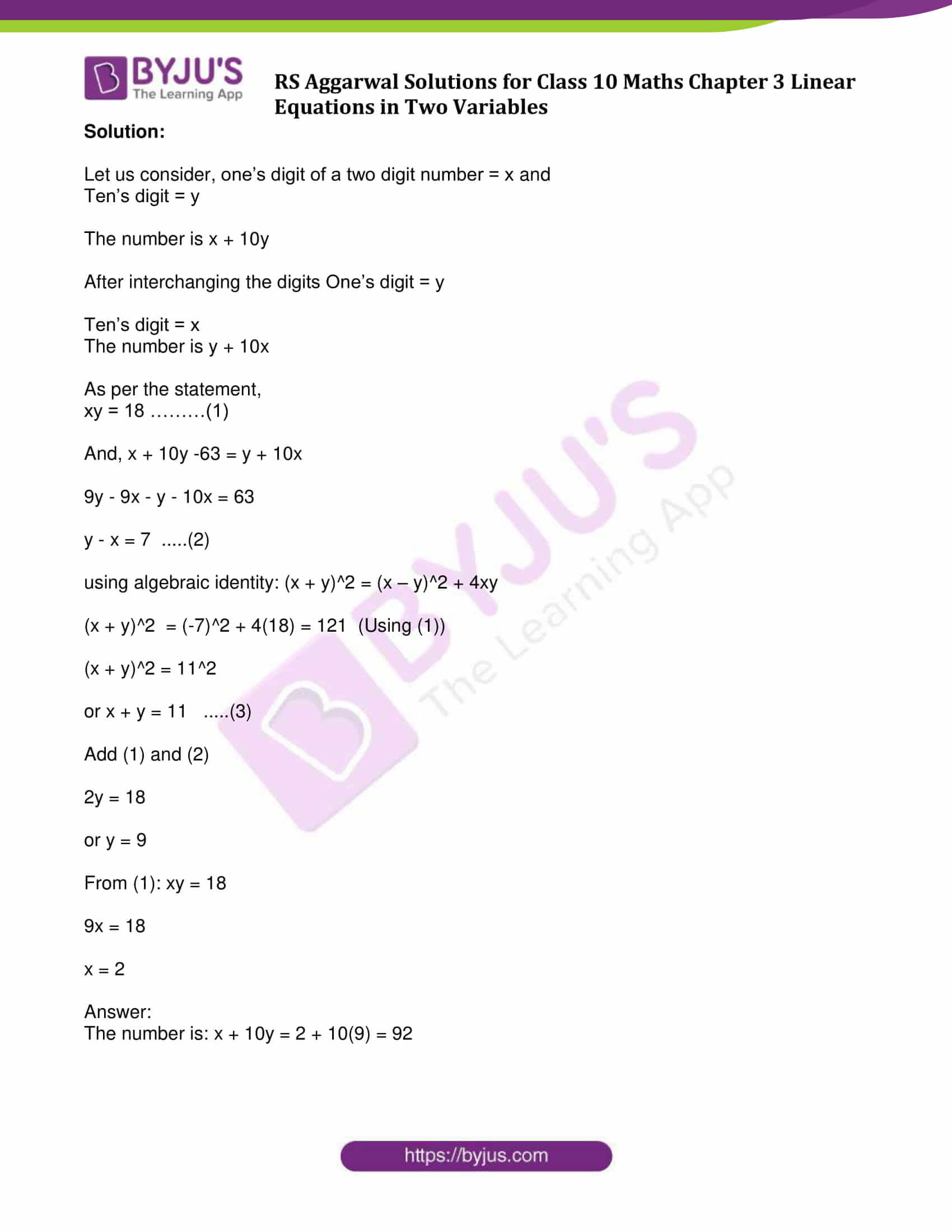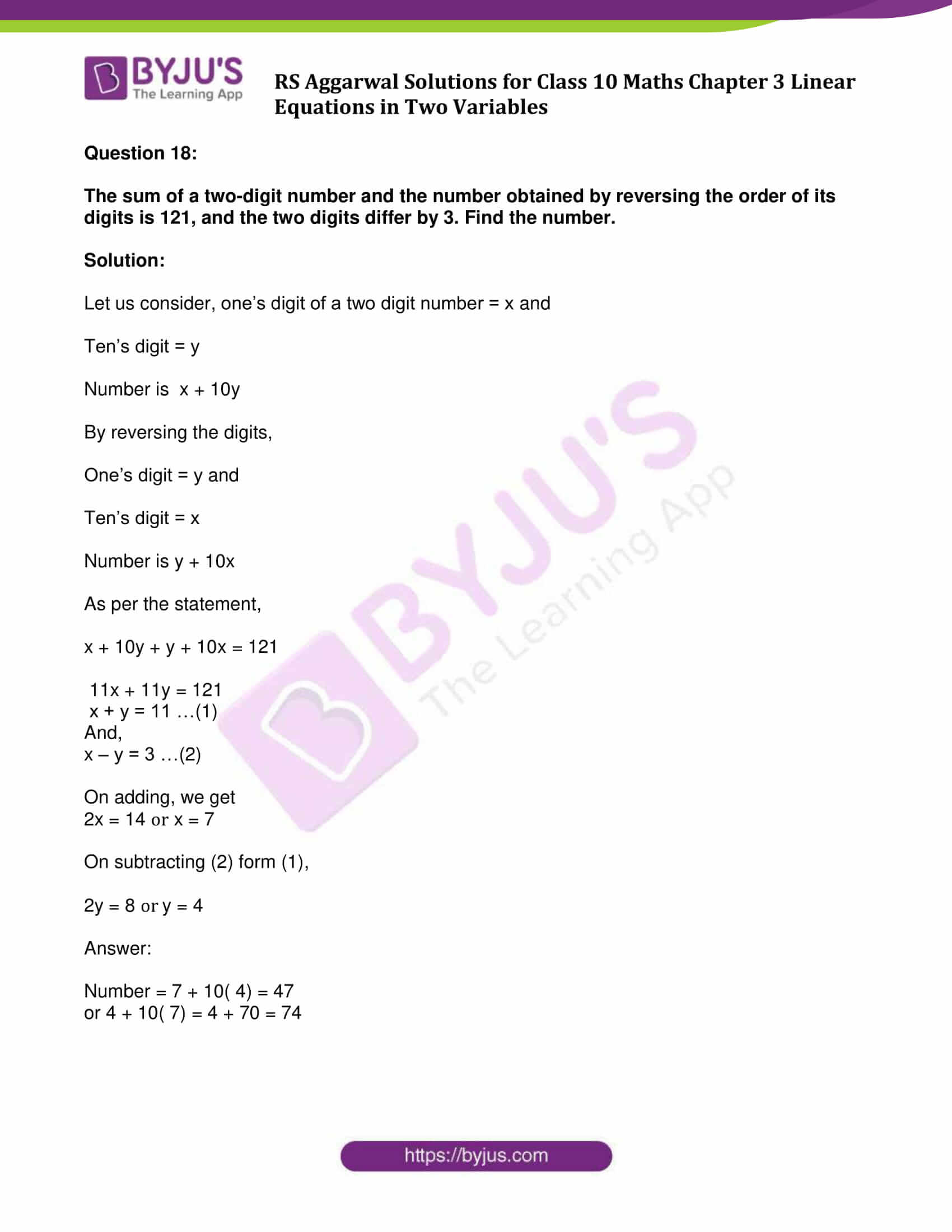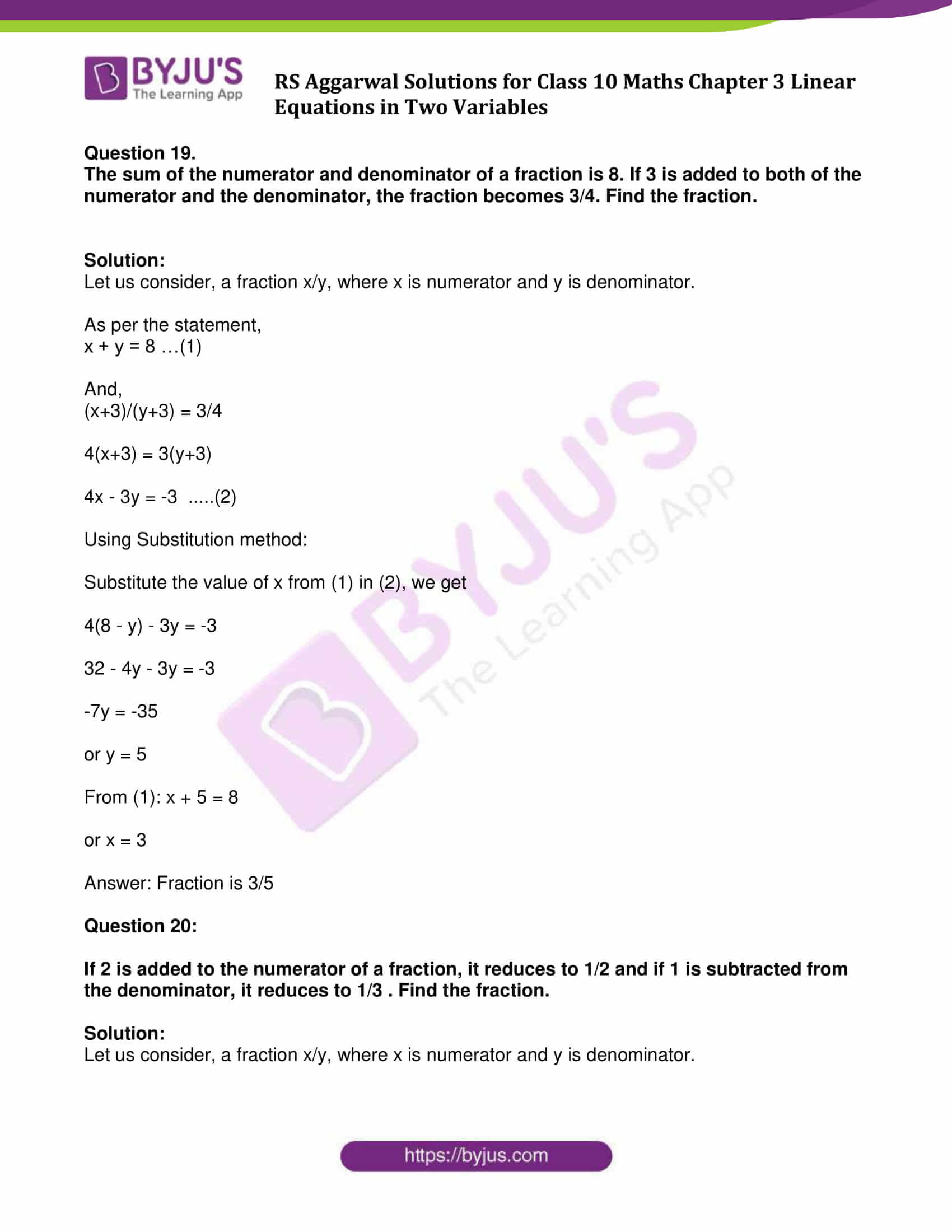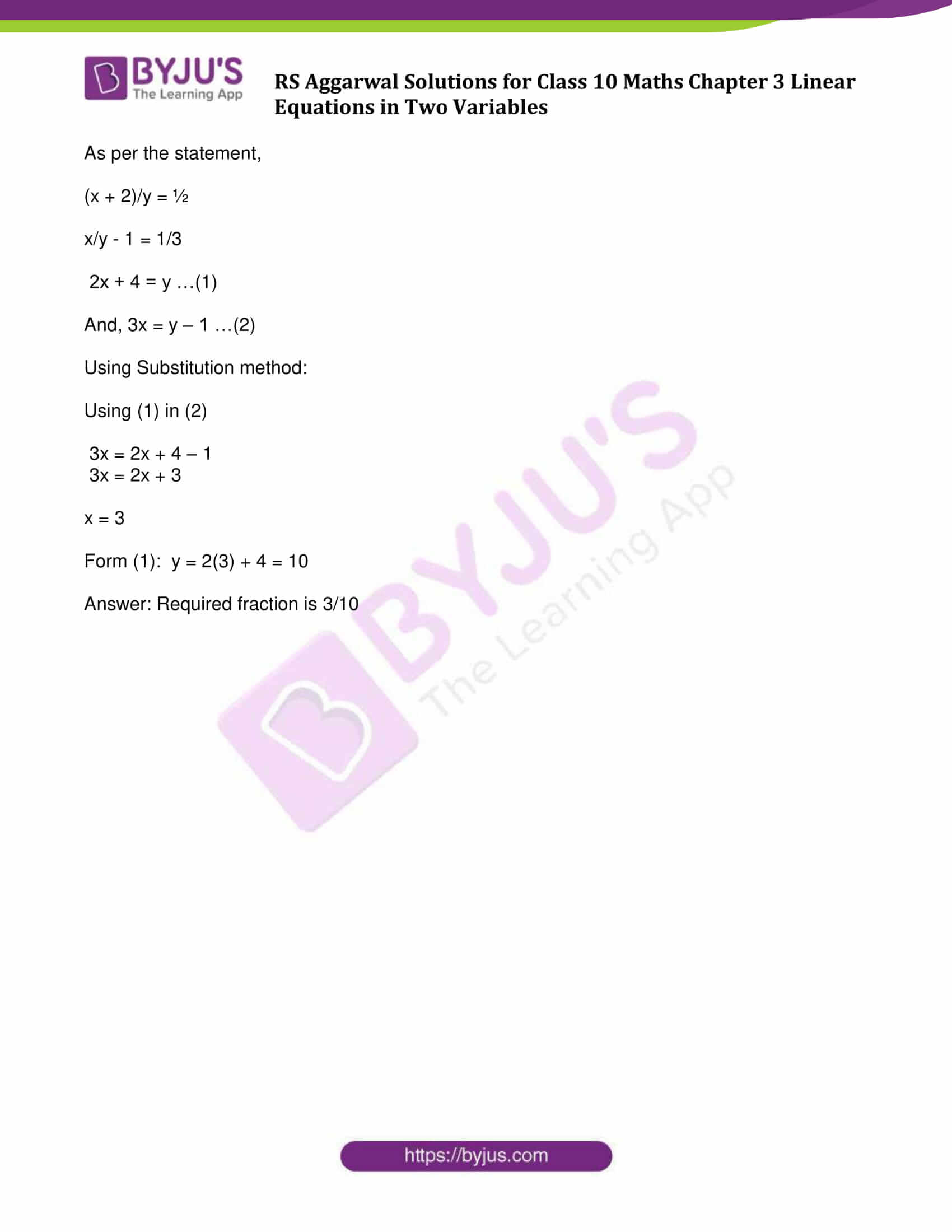## Access other exercise solutions of Class 10 Maths Chapter 3 Linear Equations in Two Variables

Exercise 3A Solutions: 29 Questions (Long Answers)

Exercise 3B Solutions: 20 Questions (Short Answers)

Exercise 3C Solutions: 13 Questions (Long Answers)

Exercise 3D Solutions: 14 Questions (Short Answers)

Exercise 3F Solutions: 10 Questions (6 Short Answers and 4 Long Answers)

## Exercise 3E Page No: 145

Question 1:

5 chairs and 4 tables together cost ₹ 5600, while 4 chairs and 3 tables together cost ₹ 4340. Find the cost of a chair and that of a table.

Solution:

Let us consider,

Cost of one chair = ₹ x and

Cost of one table = ₹ y

As per the statement,

5x + 4y = ₹ 5600 …(1)

4x + 3y = ₹ 4340 …(2)

Using elimination method:

Multiply (1) by 3 and (2) by 4. And subtract both the equations.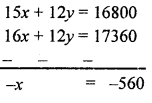So, x = 560

From (1):

5 x 560 + 4y = 5600

2800 + 4y = 5600

4y = 5600 – 2800

4y = 2800

y = 700

Cost of one chair = ₹ 560

and cost of one table = ₹ 700

Question 2:

23 spoons and 17 forks together cost ₹ 1770, while 17 spoons and 23 forks together cost ₹ 1830. Find the cost of a spoon and that of a fork.

Solution:

Let us consider,

Cost of one spoon = ₹ x and

Cost of one fork = ₹ y

As per the statement,

23x + 17y = 1770 …(1)

17x + 23y = 1830 …(2)

Adding both the equations, we get

40x + 40y = 3600

Dividing by 40,

x + y = 90 …(3)

and subtracting (2) from (1)

6x – 6y = -60

Dividing by 6,

x – y = -10 …(4)

2x = 80

or x = 40

From (3): x + y = 90

40 + y = 90

y = 50

Therefore, Cost of one spoon = ₹ 40

and cost of one fork = ₹ 50

Question 3:

A lady has only 25-paisa and 50-paisa coins in her purse. If she has 50 coins in all totalling ₹ 19.50, how many coins of each kind does she have?

Solution:

Let us consider,

Number of 25-paisa coins = x and

Number 50-paisa coins = y

Total number of coins = 50

and total amount = ₹ 19.50 or 1950 paisa

x + y = 50 …(1)

25x + 50y = 1950

x + 2y = 78 …(2)

Subtracting (1) from (2),

y = 28

And, x = 50 – y = 50 – 28 = 22

Number of 25-paisa coins = 22

and 50-paisa coins = 28

Question 4:

The sum of two numbers is 137 and their difference is 43. Find the numbers.

Solution:

Given: Sum of two numbers is 137 and difference is 43

Let us consider, first number = x and

Second number = y

x + y = 137 …..(1)

x – y = 43 ……(2)

Adding both the equations, we get

2x = 180

or x = 90

On subtracting (2) from (1),

2y = 94

y = 47

So,

First number = 90 and

Second number = 47

Question 5:

Find two numbers such that the sum of twice the first and thrice the second is 92, and four times the first exceeds seven times the second by 2.

Solution:

Let us consider, first number = x and

Second number = y

As per the statement,

2x + 3y = 92 …(1)

4x – 7y = 2 …(2)

Using elimination method:

Multiply (1) by 2 and (2) by 1, we get

4x + 6y = 184 …..(3)

4x – 7y = 2 …….(4)

Subtracting (3) from (4),

13y = 182

y = 14

From (1), 2x + 3y = 92

2x + 3 x 14 = 92

2x + 42 = 92

2x = 92 – 42 = 50

x = 25

First number = 25

Second number = 14

Question 6:

Find two numbers such that the sum of thrice the first and the second is 142, and four times the first exceeds the second by 138.

Solution:

Let us consider,

First number = x and

Second number = y

As per the statement,

3x + y=142 …(1)

4x – y = 138 …(2)

Using elimination method:

Add (1) and (2), we get

7x = 280

x = 40

From (1): 3 x 40 + y = 142

120 + y = 142

y = 142 – 120 = 22

First number = 40,

Second number = 22

Question 7:

If 45 is subtracted from twice the greater of two numbers, it results in the other number. If 21 is subtracted from twice the smaller number, it results in the greater number. Find the numbers.

Solution:

Let us consider,

First greater number = x and

Second smaller number = y

As per the statement,

2x – 45 = y …(1)

2y – 21 = x …(2)

Using Substitution method:

Substituting the value of y in (2),

2 (2x – 45) – 21 = x

4x – 90 – 21 = x

4x – x = 111

3x = 111

x = 37

From (1): y = 2 x 37 – 45 = 29

The numbers are 37 and 29.

Question 8:

If three times the larger of two numbers is divided by the smaller, we get 4 as the quotient and 8 as the remainder. If five times the smaller is divided by the larger, we get 3 as the quotient and 5 as the remainder. Find the numbers.

Solution:

Let us consider, larger number = x and

Smaller number = y

As per the statement,

3x = 4y + 8 …….(1)

5y = 3x + 5 …(2)

Using Substitution method:

Substitute the value of 3x in (2), we get

5y = 4y + 8 + 5

5y – 4y = 13

or y = 13

From (1): 3x = 4 x 13 + 8 = 60

x = 20

Larger number = 20

Smaller number = 13

Question 9:

If 2 is added to each of two given numbers, their ratio becomes 1 : 2. However, if 4 is subtracted from each of the given numbers, the ratio becomes 5 : 11. Find the numbers.

Solution:

Let us consider,

First number = x and

Second number = y

As per the statement,

(x+2)/(y+2) = 1/2

or y + 2 = 2x + 4

or y = 2x + 2 ………….(1)

And

(x-4)/(y-4) = 5/11

or 11(x-4) = 5(y – 4)

11x – 44 = 5(2x + 2) – 20 (using result of equation (1) )

11x – 44 = 10x + 10 – 20

11x – 10x = 10 – 20 + 44

or x = 34

From (1): y = 2 x 34 + 2 = 68 + 2 = 70

Numbers are 34 and 70

Question 10:

The difference between two numbers is 14 and the difference between their squares is 448. Find the numbers.

Solution:

Let us consider,

First number = x and

Second number = y

(Assume y is smaller)

As per the statement,

x – y = 14 …..(1)

and x² – y² = 448

(x + y) (x – y) = 448

(x + y) x 14 = 448

x + y = 32 ……(2)

2x = 46 or x = 23

Subtracting (1) from (2)

2y = 18 or y = 9

Numbers are 23 and 9

Question 11:

The sum of the digits of a two-digit number is 12. The number obtained by interchanging its digits exceeds the given number by 18. Find the number.

Solution:

Let us consider,

One’s digit of a two digit number = x and

Ten’s digit = y

So, the number is x + 10y

By interchanging the digits,

One’s digit = y and

Ten’s digit = x

Number is y + 10x

As per the statement,

x + y = 12 ………. (1)

y + 10x = x + 10y + 18

y + 10x – x – 10y = 18

x – y = 2 …(2)

Adding (1) and (2), we have

2x = 14 or x = 7

On subtracting (1) from (2),

2y = 10 or y = 5

Number = 7 + 10 x 5 = 57

Question 12:

A number consisting of two digits is seven times the sum of its digits. When 27 is subtracted from the number, the digits are reversed. Find the number.

Solution:

Let us consider, one’s digit of a two digit number = x and

ten’s digit = y

The number is x + 10y

After reversing the digits,

One’s digit = y

and ten’s digit = x

The number is y + 10x

As per the statement,

x + 10y – 27 = y + 10x

y + 10x – x – 10y = -27

9x – 9y = -27

x – y = -3 …(1)

Again,

7 (x + y) = x + 10y

7x + 7y = x+ 10y

7x – x = 10y – 7y

6x = 3y

2x = y …(2)

Using Substitution method:

Substituting the value of y in (1)

x – 2x = -3

-x = -3

or x = 3

From (2): y = 2(3) = 6

Number = x + 10y = 3 + 10( 6) = 63

Question 13:

The sum of the digits of a two-digit number is 15. The number obtained by interchanging the digits exceeds the given number by 9. Find the number.

Solution:

Let us consider, one’s digit of a two digit number = x and

ten’s digit = y

The number is x + 10y

After interchanging the digits,

One’s digit = y and

ten’s digit = x

The number is y + 10x

As per the statement,

x + y= 15 ………….(1)

And,

y + 10x = x + 10y + 9

y + 10x – x – 10y = 9

9x – 9y = 9

x – y = 1 …(2)

2x = 16

Or x = 8

On subtracting (2) from (1)

2y = 14

y = 7

Number = x + 10y = 8 + 10( 7) = 78

Question 14:

A two-digit number is 3 more than 4 times the sum of its digits. If 18 is added to the number, the digits are reversed. Find the number.

Solution:

Let us consider, one’s digit of the two digit number = x and

Ten’s digit = y

The number is x + 10y

By reversing the digits,

One’s digit = y and

ten’s digit = x

The number is y + 10x

Now, As per the statement,

4(x + y) + 3 = x + 10y

4x + 4y + 3 = x + 10y

3x – 6y = -3

x – 2y = -1 ……..(1)

And,

x + 10y + 18 = y + 10x

18 = y + 10x – x – 10y

9x – 9y = 18

x – y = 2 …(2)

On subtracting (1) form (2)

y = 3

Form (1): x = 2y – 1 = 2(3 ) – 1 = 5

Number = x + 10y = 5 + 10 (3) = 35

Question 15:

A number consists of two digits. When it is divided by the sum of its digits, the quotient is 6 with no remainder. When the number is diminished by 9, the digits are reversed. Find the number.

Solution:

Let us consider, ones digit of a two digit number = x and

Tens digit = y

The number is x + 10y

By reversing the digits,

One’s digit = y and ten’s digit = x

The number is y + 10x

As per the statement,

(x + 10y)/(x+y) = 6

x + 10y = 6(x + y)

5x = 4y

or x = 4/5 y …………(1)

And,

x + 10y – 9 = y + 10x

x + 10y – y – 10x = 9

-9x + 9y = 9

x – y = -1

using equation (1)

4/5 y – y = -1

y = 5

From (1): x = 4/5(5) = 4

The number is: x + 10y = 4 + 10(5) = 54

Question 16:

A two-digit number is such that the product of its digits is 35. If 18 is added to the number, the digits interchange their places. Find the number.

Solution:

Let us consider,

One’s digit of a two digit number = x and

Ten’s digit = y

The number is x + 10y

By interchanging the digits,

One’s digit = y and ten’s digit = x

The number is y + 10x

As per the statement,

xy = 35 ………(1)

And, x + 10y + 18 = y + 10x

18 = y + 10x – x – 10y

x – y = 2 …(2)

using algebraic identity: (x + y)² = (x – y)² + 4xy

(x + y)² = (2)2 + 4(35)

= 144

= (12)2

Or x + y = 12 …(3)

Subtracting (2) from (3), we get

2x =14

or x = 7

Form (1) : xy = 35

7(y) = 35

y = 5

x + 10y = 7 + 10(5) = 57

Question 17:

A two-digit number is such that the product of its digits is 18. When 63 is subtracted from the number, the digits interchange their places. Find the number.

Solution:

Let us consider, one’s digit of a two digit number = x and

Ten’s digit = y

The number is x + 10y

After interchanging the digits One’s digit = y

Ten’s digit = x

The number is y + 10x

As per the statement,

xy = 18 ………(1)

And, x + 10y -63 = y + 10x

9y – 9x – y – 10x = 63

y – x = 7 …..(2)

using algebraic identity: (x + y)^2 = (x – y)^2 + 4xy

(x + y)^2 = (-7)^2 + 4(18) = 121 (Using (1))

(x + y)^2 = 11^2

or x + y = 11 …..(3)

2y = 18

or y = 9

From (1): xy = 18

9x = 18

x = 2

The number is: x + 10y = 2 + 10(9) = 92

Question 18:

The sum of a two-digit number and the number obtained by reversing the order of its digits is 121, and the two digits differ by 3. Find the number.

Solution:

Let us consider, one’s digit of a two digit number = x and

Ten’s digit = y

Number is x + 10y

By reversing the digits,

One’s digit = y and

Ten’s digit = x

Number is y + 10x

As per the statement,

x + 10y + y + 10x = 121

11x + 11y = 121

x + y = 11 …(1)

And,

x – y = 3 …(2)

2x = 14 or x = 7

On subtracting (2) form (1),

2y = 8 or y = 4

Number = 7 + 10( 4) = 47

or 4 + 10( 7) = 4 + 70 = 74

Question 19.

The sum of the numerator and denominator of a fraction is 8. If 3 is added to both of the numerator and the denominator, the fraction becomes 3/4. Find the fraction.

Solution:

Let us consider, a fraction x/y, where x is numerator and y is denominator.

As per the statement,

x + y = 8 …(1)

And,

(x+3)/(y+3) = 3/4

4(x+3) = 3(y+3)

4x – 3y = -3 …..(2)

Using Substitution method:

Substitute the value of x from (1) in (2), we get

4(8 – y) – 3y = -3

32 – 4y – 3y = -3

-7y = -35

or y = 5

From (1): x + 5 = 8

or x = 3

Question 20:

If 2 is added to the numerator of a fraction, it reduces to 1/2 and if 1 is subtracted from the denominator, it reduces to 1/3 . Find the fraction.

Solution:

Let us consider, a fraction x/y, where x is numerator and y is denominator.

As per the statement,

(x + 2)/y = ½

x/y – 1 = 1/3

2x + 4 = y …(1)

And, 3x = y – 1 …(2)

Using Substitution method:

Using (1) in (2)

3x = 2x + 4 – 1

3x = 2x + 3

x = 3

Form (1): y = 2(3) + 4 = 10